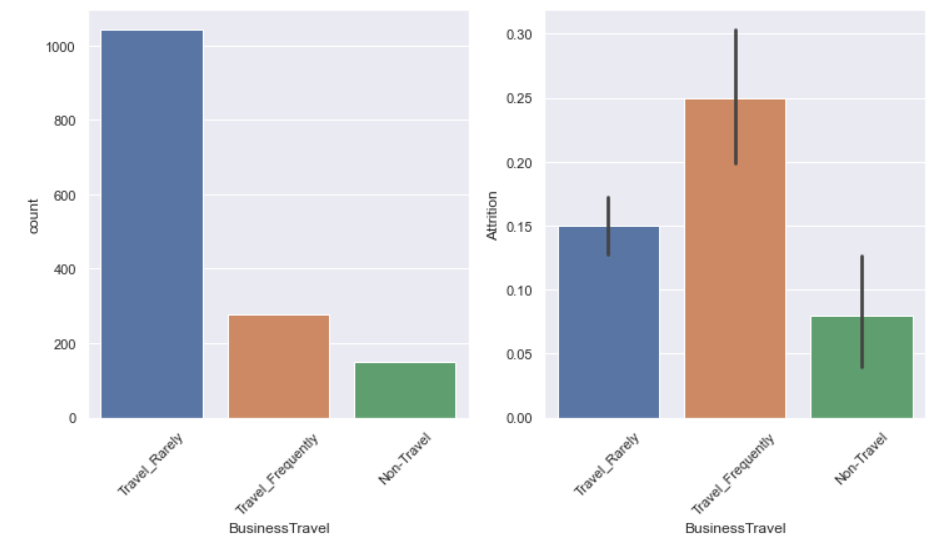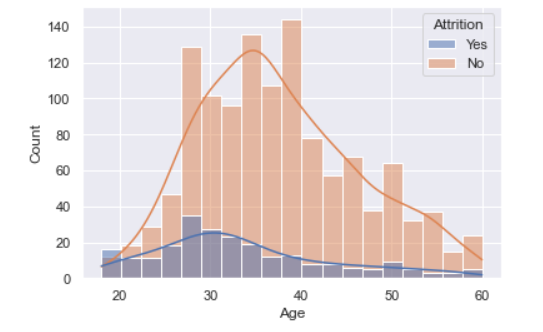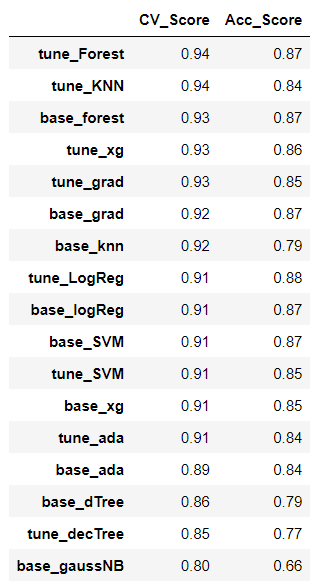# Employees Attrition Analysis — #DS001Photo by Annie Spratt on Unsplash

In this #DS001 project, I would like to do data analysis on the Employee Attrition & Performance dataset. For full code, you could check it here. So, let’s dive in.

# Problem Statements

When we are in Human Resources Department, it is a huge cost of resources if we want to hire a new employee. Not only cost you time, but also money, energy, and opportunities. So in this project, we will position ourselves in an HR Department to overcome the problem above.

# Project Goals

So in this project, I would try to explore & find 3 different aspects inside the dataset.

1. Finding the most important features
2. Training and testing the dataset
3. Finding the best model with CV score & accuracy as the key metrics

# Project Workflow

The workflow of this project consisted of 5 steps which we would explore down below.

1. Import Libraries & Dataset
2. Exploratory Data Analysis (EDA)
3. Data Preprocessing
4. Model Training & Testing
5. Final Model

# Import Libraries & Dataset

In this project, I used jupyter notebook & Python programming language to do my analysis. Firstly, we would import libraries & the dataset. The Python libraries which we needed for this project are Numpy, Pandas, Matplotlib, Seaborn, Imblearn, XGBoost, and Sklearn.

`# Setup & Import Librariesimport numpy as npimport pandas as pdimport matplotlib.pyplot as pltimport seaborn as snsfrom imblearn.over_sampling import SMOTE%matplotlib inlinesns.set()from sklearn.model_selection import GridSearchCV, train_test_splitfrom sklearn.preprocessing import StandardScalerfrom sklearn.pipeline import make_pipelinefrom sklearn.naive_bayes import GaussianNBfrom sklearn.tree import DecisionTreeClassifierfrom sklearn.linear_model import LogisticRegressionfrom sklearn.neighbors import KNeighborsClassifierfrom sklearn.svm import SVCfrom sklearn.ensemble import RandomForestClassifier, AdaBoostClassifier, GradientBoostingClassifierfrom xgboost import XGBClassifierfrom sklearn.metrics import classification_report, confusion_matrix, plot_confusion_matrix, accuracy_score# Load datasetdf = pd.read_csv('./1.2 Human_Resources.csv')cols = df.columnsdf.head()`

This dataset consists of 34 feature columns & 1 target column, which is the Attrition column.

# Exploratory Data Analysis (EDA)

Before we dive deeper into the nitty gritty of analysis, we should understand what is going on inside our dataset. Here, we would do some exploration into our dataset which I divided into several tasks.

Check for data information

`# General information of the datasetdf.info()# Check for missing values - in %agedf.isna().sum()*100/len(df)# Splitting between categorical & numerical featuresdf_cat = df.select_dtypes(exclude=np.number)df_num  = df.select_dtypes(include=np.number)# Map Attrition columndf['Attrition'] = df['Attrition'].apply(lambda x: 1 if x=="Yes" else 0)`

Fortunately, we didn’t find any missing data in the dataset, but we needed to split it into categorical features & numerical features. Notice we needed to map out data values inside ‘Attrition’ column for the analysis later.

Data Visualization

To better understand the dataset, we could implement some data visualizations. I divided this process depending on the type of feature. For categorical features, I would use the first block code and then for latter for numerical features.

`# countplot & barplot of categorical features# check the relationship between each categorical feature & Attritionfor col in df_cat.columns:    if col == 'Attrition':        sns.countplot(x=df[col])        plt.show()    else:        fig, ax = plt.subplots(1,2, figsize=(12,6))        sns.countplot(x=df[col], ax=ax)        sns.barplot(x=df[col], y=df['Attrition'], ax=ax)        for tick in ax.get_xticklabels():            tick.set_rotation(45)        for tick in ax.get_xticklabels():            tick.set_rotation(45)        plt.show()`one of examples of the output from the code block above; countplot & barplot for ‘BusinessTravel’ feature
`# Histogram & kde for numerical featuresfor col in df_num.columns:    try:        sns.histplot(kde=True, data=df, x=col, hue='Attrition')        plt.show()    except Exception as e:        print (col)        print (e)`one of examples of the output from the code block above; hisplot for ‘Age’ feature

From the code blocks above, we got some insights.

• The “Attrition” in the dataset is around 17%
• For the ‘JobRole’ feature of ‘Sales Representative’, they will have a higher chance of Attrition
• For the ‘MaritalStatus’ feature of ‘Single’, they will have a higher chance of Attrition
• For the ‘Overtime’ feature of ‘Yes’, they will have a higher chance of Attrition
• People with ‘Age’ of around ‘30’, or ‘MontlyIncome’ around 2500, or ‘PercentSalaryHike’ of 12–14%, or ‘YearsAtCompany’ is still low have a higher chance of Attrition

And then for numerical features, I got some questions about how differ the Attrition of Yes & No for each numerical feature, so you could write this block of code.

`# Stats Descriptive between Yes & No Attrition for all features in numerical dataset# columns name, we use it latercol_desc = []for col in list(df_num[df['Attrition'] == 'No'].describe().T.columns):    col_desc.append(col+'_Y')for col in list(df_num[df['Attrition'] == 'No'].describe().T.columns):    col_desc.append(col+'_N')desc_df = pd.concat([df_num[df['Attrition'] == 'Yes'].describe().T, df_num[df['Attrition'] == 'No'].describe().T],          axis=1)desc_df.columns = col_desccol_desc_diff = []for col in list(df_num[df['Attrition'] == 'No'].describe().T.columns):    col_desc_diff.append(col+'_D')# difference in %age between Yes & No of "Attrition" featuresfor col in col_desc_diff:    desc_df[col] = np.abs((desc_df[(col[:-1]+'Y')] - desc_df[(col[:-1]+'N')])/desc_df[(col[:-1]+'N')])desc_df[['mean_D','std_D','50%_D']].sort_values('mean_D', ascending=False)`

The result is shown in the picture below, you could see that some features really have a big difference between Yes Attrition & No Attrition.

Next, we wanted to know about the correlation among features.

`plt.figure(figsize=(15,15))sns.heatmap(np.round(df.drop(['StandardHours','EmployeeCount'], axis=1).corr(),2), annot=True)`

From the heatmap, we found that some of features has high correlation, so I decided to drop some of them.

`# Dropped uninformative features: Over18, EmployeeCount, EmployeeNumber, StandardHours# Collinear features: MontlyIncome & JobLevel, TotalWorkingYears & JobLevel, TotalWorkingYears & MontlyIncome,# YearsAtCompany & YearsWithCurrManager, YearsAtCompany & YearsInCurrentRole# Dropped collinear features: JobLevel, TotalWorkingYears, YearsAtCompanydf_num.drop(['JobLevel','TotalWorkingYears','YearsAtCompany', 'EmployeeCount','EmployeeNumber','StandardHours'], axis=1,inplace=True)df_cat.drop('Over18', axis=1,inplace=True)`

I guessed that’s it for EDA. EDA is basically a never-ending process if you want to explore more & more.

# Model Training

Before we trained our model, we needed to convert categorical features into numeric, so the ML algorithm could train the dataset.

`#### drop_first to avoid collineardf_cat_dummy = pd.get_dummies(df_cat, drop_first=True)# Concat numerical & categorical featuresdf_dummy = pd.concat([df_cat_dummy, df_num], axis=1)df_dummy.head()`

Find the most important features

Firstly, our goal was to find the most important features which influenced the Attrition of Employees. Here, I used Random Forest Algorithm to find them

`X = df_dummy.drop(['Attrition'], axis=1)y = df_dummy['Attrition']X_train, X_test, y_train, y_test = train_test_split(X, y, test_size=0.3, random_state=101)# Balance Attrition with SMOTEsmote = SMOTE(random_state = 101)X_train, y_train = smote.fit_resample(X_train, y_train)# Scale all featuresscaler = StandardScaler()scaled_X_train = scaler.fit_transform(X_train)scaled_X_test = scaler.transform(X_test)# Training modelmodel = RandomForestClassifier(n_estimators=600, random_state=101)model.fit(scaled_X_train, y_train)importance = pd.DataFrame({'feature':X_train.columns, 'importance': np.round(model.feature_importances_,3)})importance = importance.sort_values('importance', ascending=False).set_index('feature')# Plot the important featuresimportance.plot(kind='bar', rot=90, figsize=(18,6))plt.show()`

Here are the top 5 of the most important features which actually make sense if they influence employee attrition.

1. Overtime
2. Monthly Income
3. Marital Status
4. Age
5. Job Involvement

Training & Testing the dataset

Here I divided the process of training & testing the dataset into 2 different processes, there were:

1. Training & Testing without tuning parameters (Default)
2. Training & Testing with tuning parameters.

I called the first process a baseline. We would try different kinds of ML models, there are Gaussian Naive Bayes, Decision Tree, Random Forest, SVM, KNN, AdaBoost, Gradient Boost, XGBoost, Logistic Regression

Firstly, I defined an empty dictionary that would store a testing performance for each model & I defined a function to do the training & testing process.

`result = {}def model_grid (X_train, X_test, y_train, y_test, pipe, param_grid, model_name):        grid = GridSearchCV(pipe, param_grid, cv=10)    grid.fit(X_train, y_train)        cv_score = np.round(grid.best_score_, 2)        print(f'cv score = {cv_score}\n')    print(f'best param = {grid.best_params_}')        y_hat = grid.predict(X_test)    acc_score = np.round(accuracy_score(y_test, y_hat), 2)        print(classification_report(y_test, y_hat))    result[model_name] = [cv_score, acc_score]`

For the baseline model (w/o tuning process), we just needed to call the function like this code below and then the function would store the performance metrics into the ‘result’ dictionary. Below is one of the block code examples for an only Decision Tree model, check my repo for all ML models.

`pipe = make_pipeline(StandardScaler(), DecisionTreeClassifier(random_state=101))param_grid = {}model_grid(X_train, X_test, y_train, y_test, pipe, param_grid, 'base_dTree')`

And then for models for which I did tuning parameters, we just needed to call the block code below. Below is one of the block code examples for an only Random Forest model, check my repo for all ML models.

`pipe = make_pipeline(StandardScaler(), RandomForestClassifier(random_state=101))param_grid = {'randomforestclassifier__n_estimators':[100,110,120,130,140,150],              'randomforestclassifier__max_depth':[9,10,11,12,13],             'randomforestclassifier__max_features' : ['sqrt', 'log2']}model_grid(X_train, X_test, y_train, y_test, pipe, param_grid, 'tune_Forest')`

Finally, we could call the ‘result’ to see the result of all training & testing processes for all models.

`pd.DataFrame(result, index=['CV_Score','Acc_Score']).T.sort_values(['CV_Score','Acc_Score'], ascending=False)`The final result of training & testing processes for all ML models

# Conclusion

From the result above we could see that the tuned Random Forest model had the highest scores. For my further work, I could refine the model performances by doing some Refine GridSearch methods or implementing some Neural Network or Deep Learning models.

I hope you enjoy my work, peace out …!

--

--